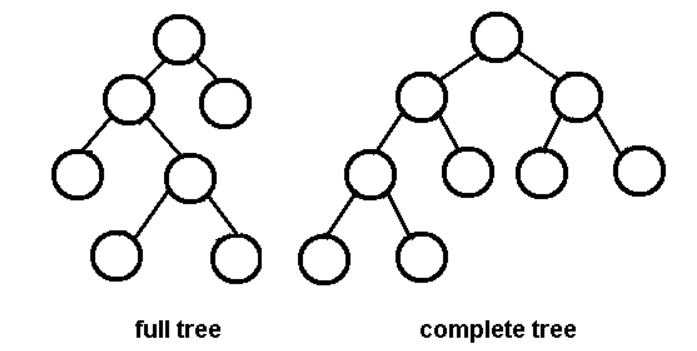# Binary Tree & Binary Search Tree

This is the summary of binary tree and binary search tree part in MAW Chapter 4.

## Concept

• A binary tree is a tree in which no node can have more than two children.
• An important application of binary trees is binary search tree: for every node, $$X$$, in the tree, the values of all the keys in its left subtree are smaller than the key value in $$X$$, and the values of all the keys in its right subtree are larger than the key value in $$X$$ (BST-property).

## Classification of binary trees

• A full binary tree (proper binary tree or 2-tree) is a binary tree in which each node has exactly zero or two children.

Note

A full node in a binary tree is a node that has exactly two non-null children.

• A complete binary tree is a binary tree, which is completely filled, with the possible exception of the bottom level, which is filled from left to right.• A perfect binary tree: A binary tree in which all internal nodes have exactly two children and all leaves are at the same level. It has property: each level has exactly twice as many nodes as the previous level (since each internal node has exactly two children).• A balance binary tree: a binary tree structure in which the left and right subtrees of every node differ in height by no more than 1. Yes, AVL tree definition.

## Properties

• The average depth of binary tree is $$O(\sqrt{n})$$
• The height of balance binary tree is $$O(\log n)$$
• A complete binary tree on $$n$$ nodes has height $$\lfloor \log n \rfloor$$
• A binary tree of $$N$$ nodes, there are $$N+1$$ NULL pointers representing children (MAW 4.4)
• The maximum number of nodes in a binary tree of height $$H$$ is $$2^{H+1}-1$$ (MAW 4.5)
• A perfect binary tree of height $$H$$ contains exactly $$2^{H+1}-1$$ nodes, of which $$2^H$$ are leaves.
• The number of full nodes plus one is equal to the number of leaves in a nonempty binary tree
• The average depth of a node in a binary search tree constructed from random data is $$O(\log n)$$
• The average of height of a random binary search tree is $$O(\log n)$$ (i.e., $$E[h] = O(\log n)$$) (MAW 4.14)
• All the basic operations find, findMin, findMax, insert, and delete $$O(H)$$ time, where $$H$$ is the height of the tree.
• worst case: height $$H = n - 1$$
• base case: height $$H = \log N$$, where the tree is a complete binary tree.
• Randomly built binary search trees:
• The average height is much closer to the best case.
• Little is known about the average height when both insertion and deletion are used.
• characteristics
• Keys inserting in random order into an initially empty tree.
• Each of the $$n!$$ permutations of the input keys is equally likely.

## Selected Proofs

Let's prove "The number of full nodes plus one is equal to the number of leaves in a nonempty binary tree" using induction:

• Let $$n$$ be the number of full nodes and $$m$$ be the number of leaves in a nonempty binary tree. Then, we have $$n + 1 = m$$.

• Base case: $$n = 0$$, since the binary tree is nonempty, we have the degenerated binary tree with $$m = 1$$.
• Recursion: Suppose the claim holds for $$n$$ (i.e., $$n + 1 = m$$. We want to show that the claim also holds for $$n+1$$. There are two cases we need to consider:
• We add two leaves to form one extra full node. In this case, we have $$m - 1 + 2 = m+1$$ leaves. Thus, we have $$m+1 - (n+1) = n+1+1 - (n+1) = 1$$. The claim holds.
• We add one leaf to form one extra full node. In this case, we have $$m + 1$$ leaves. Thus, we have $$m+1 - (n+1) = 1$$. The claim holds.